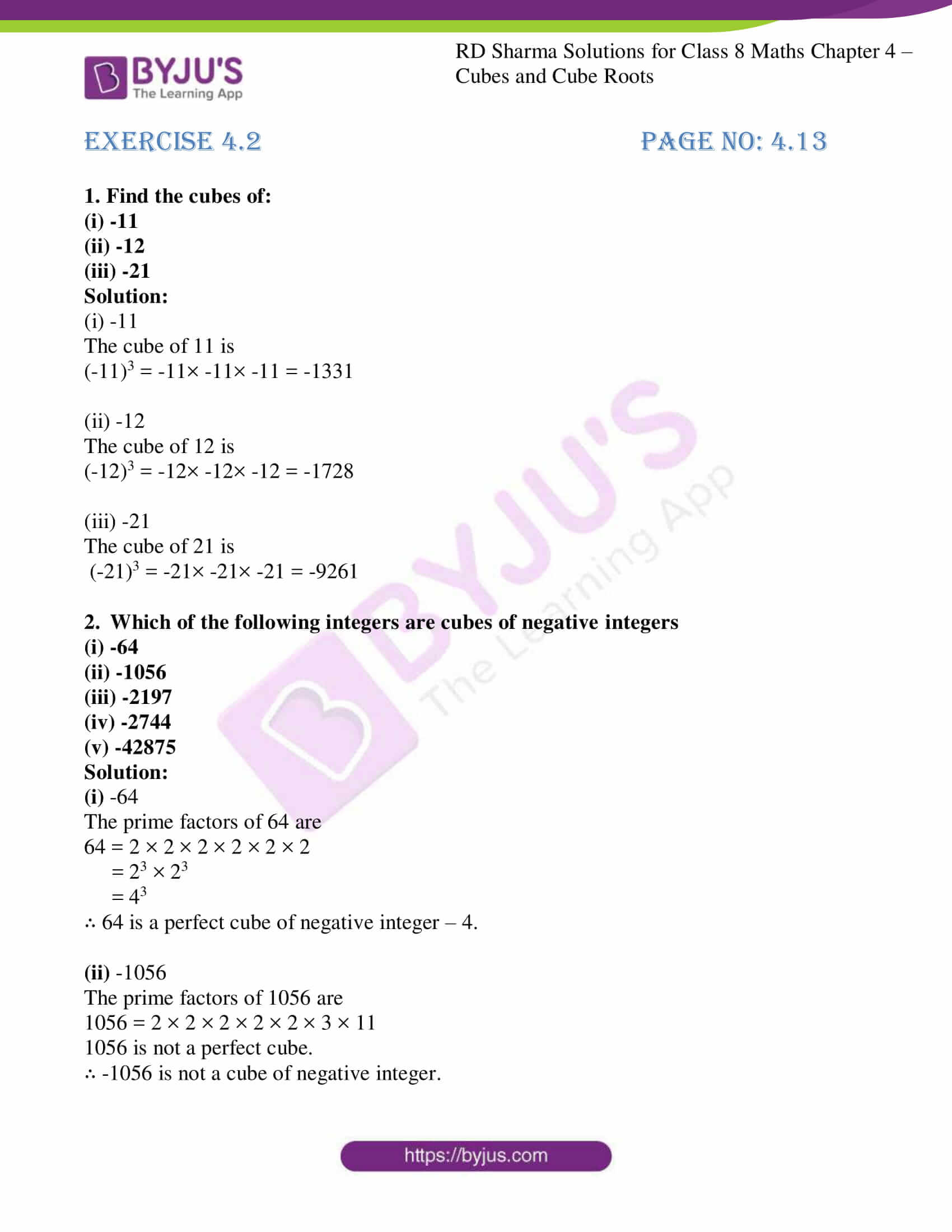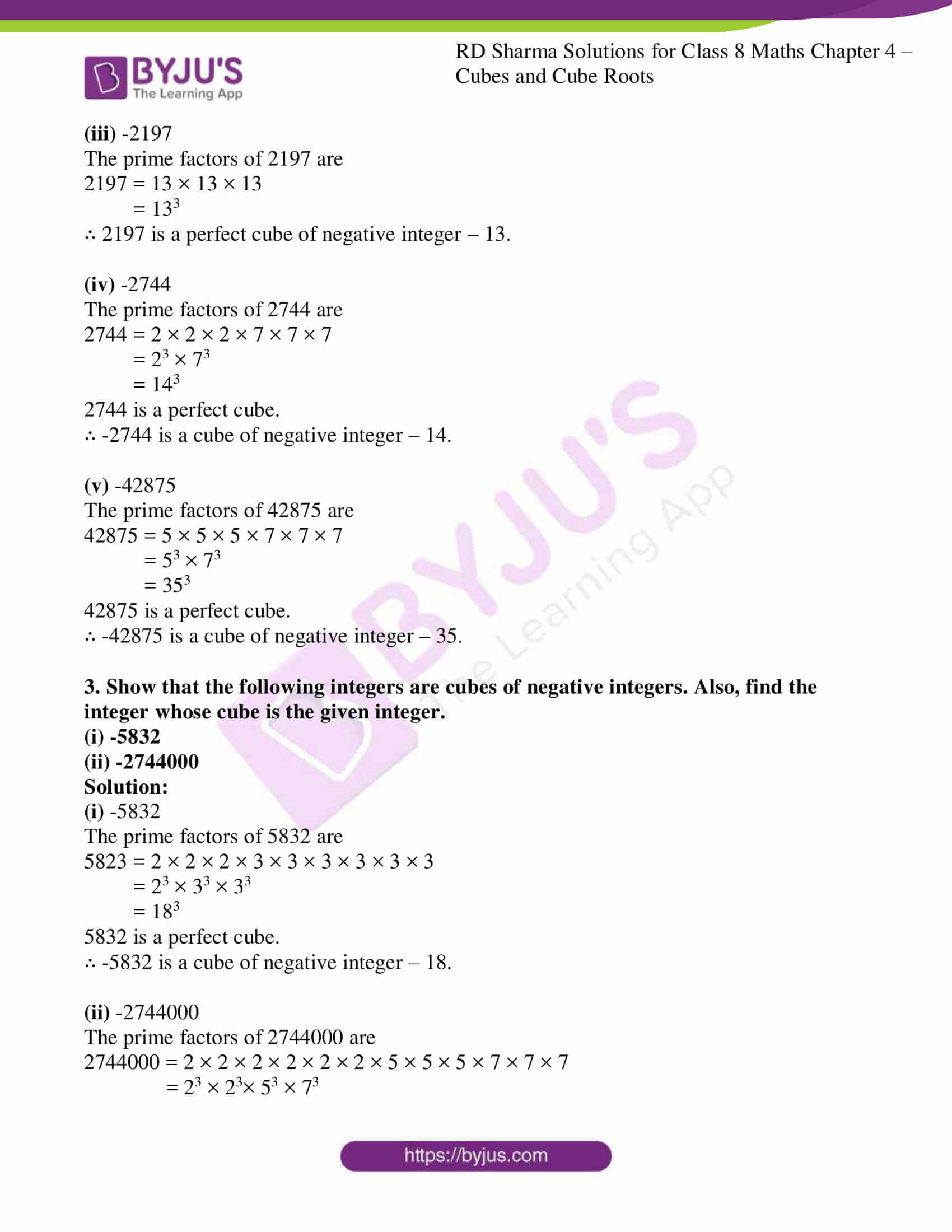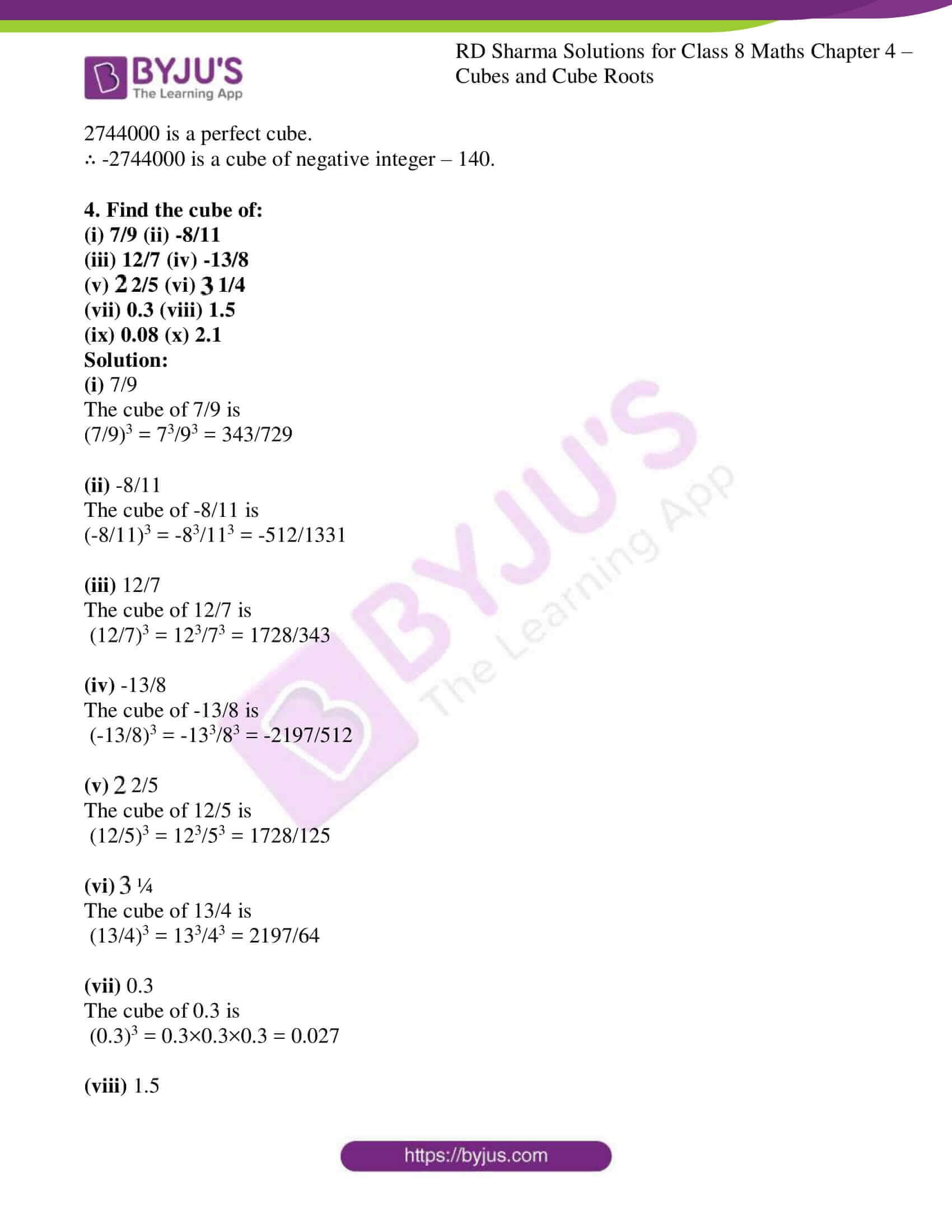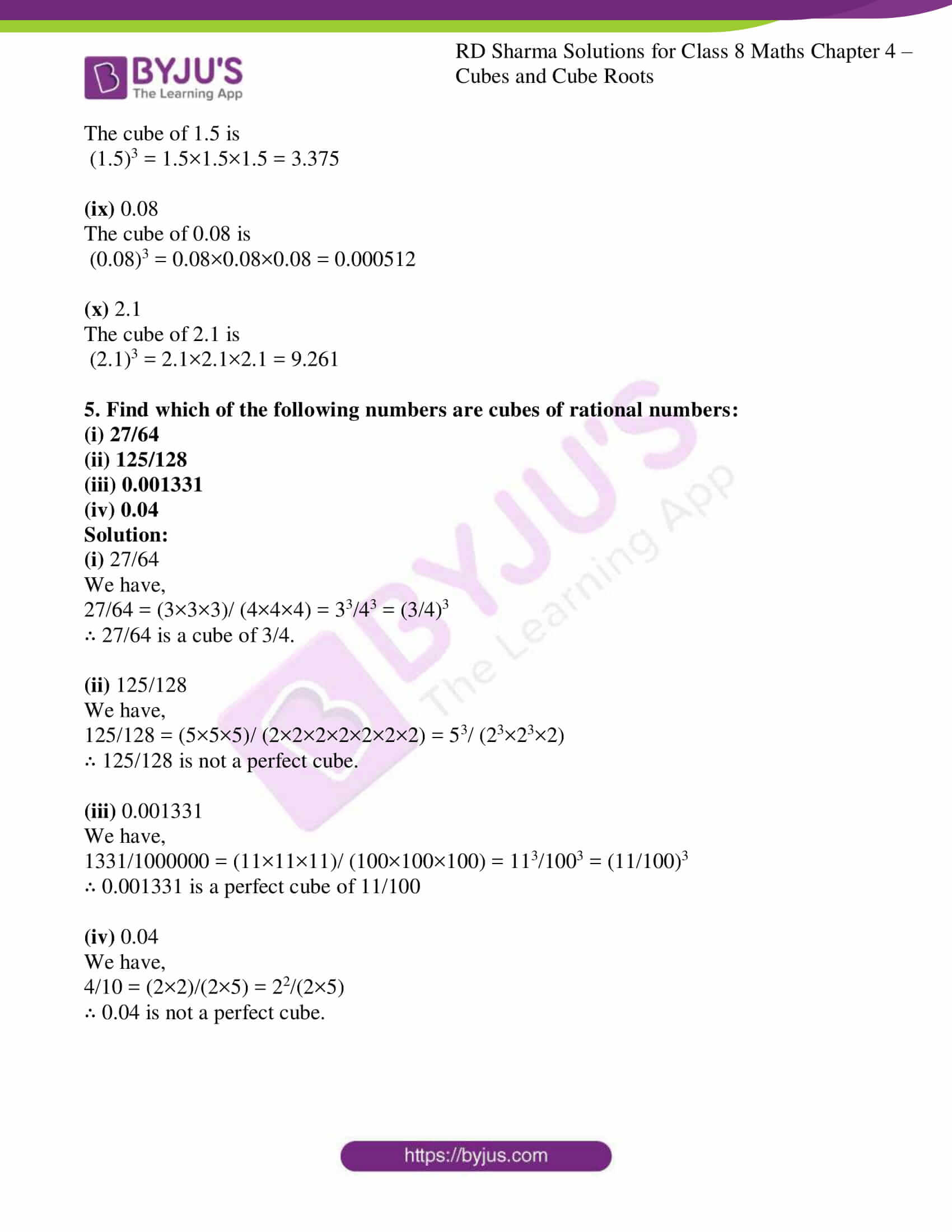# RD Sharma Solutions Class 8 Maths Chapter 4 Cubes And Cube Roots Exercise 4.2

Students can refer and download RD Sharma Class 8 Maths from the links provided below. In Exercise 4.2 of RD Sharma Class 8 Maths Chapter 4 Cubes and Cube Roots, we shall discuss problems based on cubes of negative integers and cubes of rational numbers. RD Sharma Class 8 Solutions are customized and derived in a step by step approach by our expert team, which helps students in gaining proficiency over the concepts.

## Download PDF of RD Sharma Solutions for Class 8 Maths Exercise 4.2 Chapter 4 Cubes and Cube Roots### Access Answers to RD Sharma Solutions for Class 8 Maths Exercise 4.2 Chapter 4 Cubes and Cube Roots

1. Find the cubes of:
(i) -11
(ii) -12
(iii) -21

Solution:

(i) -11

The cube of 11 is

(-11)3 = -11× -11× -11 = -1331

(ii) -12

The cube of 12 is

(-12)3 = -12× -12× -12 = -1728

(iii) -21

The cube of 21 is

(-21)3 = -21× -21× -21 = -9261

2. Which of the following integers are cubes of negative integers
(i) -64
(ii) -1056
(iii) -2197
(iv) -2744
(v) -42875

Solution:

(i) -64

The prime factors of 64 are

64 = 2 × 2 × 2 × 2 × 2 × 2

= 23 × 23

= 43

∴ 64 is a perfect cube of negative integer – 4.

(ii) -1056

The prime factors of 1056 are

1056 = 2 × 2 × 2 × 2 × 2 × 3 × 11

1056 is not a perfect cube.

∴ -1056 is not a cube of negative integer.

(iii) -2197

The prime factors of 2197 are

2197 = 13 × 13 × 13

= 133

∴ 2197 is a perfect cube of negative integer – 13.

(iv) -2744

The prime factors of 2744 are

2744 = 2 × 2 × 2 × 7 × 7 × 7

= 23 × 73

= 143

2744 is a perfect cube.

∴ -2744 is a cube of negative integer – 14.

(v) -42875

The prime factors of 42875 are

42875 = 5 × 5 × 5 × 7 × 7 × 7

= 53 × 73

= 353

42875 is a perfect cube.

∴ -42875 is a cube of negative integer – 35.

3. Show that the following integers are cubes of negative integers. Also, find the integer whose cube is the given integer.
(i) -5832
(ii) -2744000

Solution:

(i) -5832

The prime factors of 5832 are

5823 = 2 × 2 × 2 × 3 × 3 × 3 × 3 × 3 × 3

= 23 × 33 × 33

= 183

5832 is a perfect cube.

∴ -5832 is a cube of negative integer – 18.

(ii) -2744000

The prime factors of 2744000 are

2744000 = 2 × 2 × 2 × 2 × 2 × 2 × 5 × 5 × 5 × 7 × 7 × 7

= 23 × 23× 53 × 73

2744000 is a perfect cube.

∴ -2744000 is a cube of negative integer – 140.

4. Find the cube of:
(i) 7/9 (ii) -8/11
(iii) 12/7 (iv) -13/8
(v) $$2\frac{2}{5}$$ (vi) $$3\frac{1}{4}$$ (vii) 0.3 (viii) 1.5
(ix) 0.08 (x) 2.1

Solution:

(i) 7/9

The cube of 7/9 is

(7/9)3 = 73/93 = 343/729

(ii) -8/11

The cube of -8/11 is

(-8/11)3 = -83/113 = -512/1331

(iii) 12/7

The cube of 12/7 is

(12/7)3 = 123/73 = 1728/343

(iv) -13/8

The cube of -13/8 is

(-13/8)3 = -133/83 = -2197/512

(v) $$2\frac{2}{5}$$

The cube of 12/5 is

(12/5)3 = 123/53 = 1728/125

(vi) $$3\frac{1}{4}$$

The cube of 13/4 is

(13/4)3 = 133/43 = 2197/64

(vii) 0.3

The cube of 0.3 is

(0.3)3 = 0.3×0.3×0.3 = 0.027

(viii) 1.5

The cube of 1.5 is

(1.5)3 = 1.5×1.5×1.5 = 3.375

(ix) 0.08

The cube of 0.08 is

(0.08)3 = 0.08×0.08×0.08 = 0.000512

(x) 2.1

The cube of 2.1 is

(2.1)3 = 2.1×2.1×2.1 = 9.261

5. Find which of the following numbers are cubes of rational numbers:
(i) 27/64
(ii) 125/128
(iii) 0.001331
(iv) 0.04

Solution:

(i) 27/64

We have,

27/64 = (3×3×3)/ (4×4×4) = 33/43 = (3/4)3

∴ 27/64 is a cube of 3/4.

(ii) 125/128

We have,

125/128 = (5×5×5)/ (2×2×2×2×2×2×2) = 53/ (23×23×2)

∴ 125/128 is not a perfect cube.

(iii) 0.001331

We have,

1331/1000000 = (11×11×11)/ (100×100×100) = 113/1003 = (11/100)3

∴ 0.001331 is a perfect cube of 11/100

(iv) 0.04

We have,

4/10 = (2×2)/(2×5) = 22/(2×5)

∴ 0.04 is not a perfect cube.